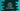# 4 different Kotlin programs to add two numbers## Kotlin program to add two numbers:

This post will show you how to add two numbers in Kotlin. The addition of two numbers or finding the sum of two numbers is similar to any other programming language in Kotlin. We will learn two different ways to write this program:

• By using predefined constant numbers
• By taking the numbers as inputs from the user

We have to use the addition operator, + to add two numbers. Let’s learn how to write it in code.

### Example 1: Kotlin program to add two numbers with predefined values:

In this program, we will use two predefined numbers and the program will find the sum of these numbers.

``````fun main() {
val firstNumber = 11;
val secondNumber = 23;

val sum = firstNumber + secondNumber;

print("\$firstNumber + \$secondNumber = \$sum")
}``````

Here,

• firstNumber is the variable to hold the first number and secondNumber is the variable to hold the second number.
• These numbers are added and the sum is assigned to the variable sum.
• The last line is printing the result to the user.

If you run this program, it will print the below result:

``11 + 23 = 34``

### Example 2: Kotlin program to add two numbers with user input values:

We can also take the numbers as inputs from the user. The program will ask the user to enter the numbers. It will read the numbers, assign these to different variables and print the result.

Below is the complete program:

``````import java.util.*

fun main() {
val scanner = Scanner(System.`in`)

println("Enter the first number: ")
val firstNumber = scanner.nextInt()

println("Enter the second number: ")
val secondNumber = scanner.nextInt()
val sum = firstNumber + secondNumber;

print("\$firstNumber + \$secondNumber = \$sum")
}``````

In this method,

• The scanner is a Scanner object to read the user input.
• It asks the user to enter the numbers one by one. To read the user input numbers, it is using the nextInt() method of scanner. The first number is assigned to firstNumber variable and the second number to secondNumber variable. The nextInt() method reads the next user input value as an integer.
• The sum of the numbers are calculated and stored in the sum variable.

If you run this program, it will print output as like below:

``````Enter the first number:
12
Enter the second number:
13
12 + 13 = 25

Enter the first number:
243
Enter the second number:
443
243 + 443 = 686``````

### Example 3: Kotlin program to add two floating point numbers with user input values:

The above program works for integers. If user enters any float values, we will have to use nextFloat instead of nextInt.

The following program uses the nextFloat method of the scanner class to read the user input value as float.

``````import java.util.*

fun main() {
val scanner = Scanner(System.`in`)

println("Enter the first number: ")
val firstNumber = scanner.nextFloat()

println("Enter the second number: ")
val secondNumber = scanner.nextFloat()
val sum = firstNumber + secondNumber;

print("\$firstNumber + \$secondNumber = \$sum")
}``````

It will print output as like below:

``````Enter the first number:
443
Enter the second number:
244
443.0 + 244.0 = 687.0

Enter the first number:
223.65
Enter the second number:
998.78
223.65 + 998.78 = 1222.43``````

### Method 4: Kotlin program to add N user input numbers:

Let’s write a program that will take N different numbers as input from the user and find the sum of all the user-input numbers.

``````import java.util.*

fun main() {
val scanner = Scanner(System.`in`)
var currentNumber = 0
var sum = 0

while (true) {
println("Enter a number, Enter -1 to stop: ")

currentNumber = scanner.nextInt()
if (currentNumber == -1) {
break
}
sum += currentNumber
}

print("Sum of the numbers = \$sum")
}``````

Here, the while loop will run for an infinite amount of time. On each iteration, it asks the user to enter a number. If the user enters -1, the control will exit from the loop. Else, it will add that number to the current value of sum. Once the loop ends, it prints the value of sum, i.e. the sum of all the numbers the user has entered.

It will print output as below: